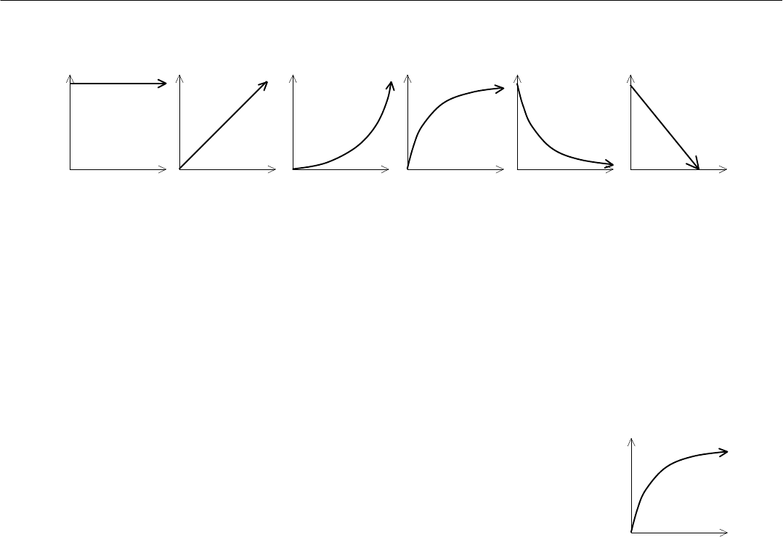Study Guides (400,000)
US (230,000)
UH (60)
PHYS (30)
All (30)
Midterm

# Hawaii PHYS 151 exam151m1-08sprExam

Department
Physics
Course Code
PHYS 151
Professor
All
Study Guide
Midterm

This preview shows page 1. to view the full 5 pages of the document.Physics 151 Roster No.:
February 13, 2008
Score:
Midterm Exam #1, Part A
Exam time limit: 50 minutes. You may use a calculator and both sides of ONE sheet of notes, handwritten
only. Closed book; no collaboration. Ignore friction and air resistance in all problems, unless told otherwise.
Part A: For each question, fill in the letter of the one best answer on your bubble answer sheet.
Physical constants: g = 9.80 m/s2
(2 pts. each) Convert the following quantities into the given units:
1. 430 km = cm
A. 4.3 × 10–7 cm D. 4.3 × 105 cm
B. 4.3 × 10–5 cm E. 4.3 × 107 cm
C. 4.3 × 10–3 cm
2. 3.5 mm/s = m/min
A. 0.21 m/min D. 2.1 × 103 m/min
B. 2.1 m/min E. 2.1 × 104 m/min
C. 2.1 × 102 m/min
3. 7.5 × 10–4 km2 = m2
A. 0.075 m2 D. 7.5 × 104 m2
B. 7.5 m2 E. 7.5 × 106 m2
C. 750 m2
4. (1 pt.) How many significant figures does the value “4.0050 × 106 kg” have?
A. 2 D. 5
B. 3 E. 6
C. 4
5. (2 pts.) How many megabytes are in one terabyte?
A. 103 D. 1012
B. 106 E. 1015
C. 109

Unlock to view full version

Only page 1 are available for preview. Some parts have been intentionally blurred.A 110-kg basketball player can jump a vertical distance of 1.3 meters (approx. 4 feet). For this entire problem,
assume that the athlete has NO horizontal motion. (Ignore air resistance.)
6. (2 pts.) What must be the player’s initial vertical velocity as he leaves the ground?
A. 3.4 m/s D. 9.2 m/s
B. 5.0 m/s E. 11 m/s
C. 7.6 m/s
7. (1 pt.) At the moment the athlete reaches his maximum height, which of the following is/are equal to zero?
A. his velocity
B. his speed
C. his acceleration
D. A & B only
E. all of the above
8. (2 pts.) How much total time elapses during his entire jump, from take-off to landing?
A. 0.78 s D. 1.6 s
B. 1.0 s E. 1.9 s
C. 1.3 s
9. (1 pt.) Suppose that a smaller, 75-kg athlete jumps with the same initial velocity as the 110.-kg athlete does.
Which one of the following is TRUE?
A. The 75-kg athlete will reach a greater maximum height.
B. The 75-kg athlete will reach a lower maximum height.
C. The 75-kg athlete will be in the air for a longer period of time before landing.
D. The 75-kg athlete will be in the air for a shorter period of time before landing.
E. None of the above.
Six different cars move along one-dimensional tracks. The following six graphs represent their VELOCITIES as
functions of time:
10. (1 pt.) Which of the above car(s) has a constant speed?
A. (1) only D. (2) & (6) only
B. (2) only E. (1), (2) & (6)
C. (6) only
11. (1 pt.) Which of the above car(s) has a constant negative acceleration?
A. (4) only D. (5) & (6) only
B. (5) only E. (4), (5) & (6)
C. (6) only
12. (1 pt.) Which of the above car(s) is slowing down?
A. (4) only D. (5) & (6) only
B. (5) only E. (4), (5) & (6)
C. (6) only
13. (2 pts.) Which of the above car(s) has a displacement graph roughly like this one:
A. (4) only D. (5) & (6) only
B. (5) only E. (4), (5) & (6)
C. (6) only
Δx
t
(1)
(2)
(3)
(4)
(5)
(6)
t
t
t
t
t
t
v
v
v
v
v
v